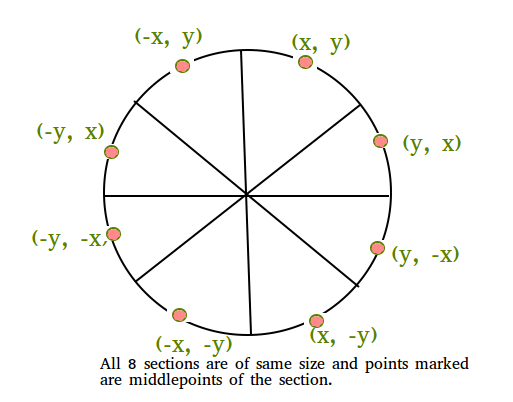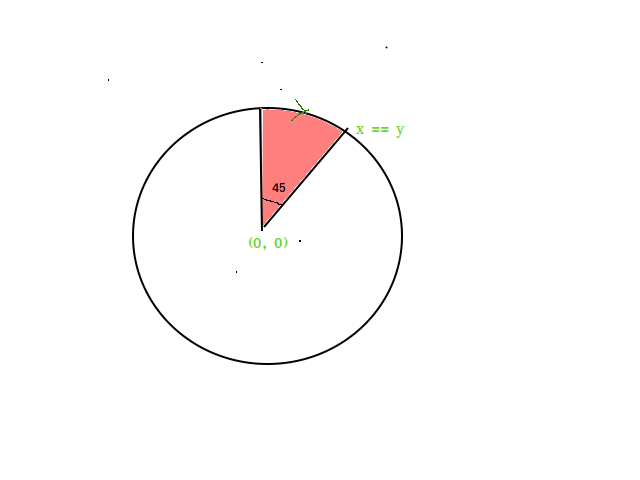# Neighbors of a point on a circle using Bresenham’s algorithm

• Last Updated : 12 Jul, 2021

Given a center of a circle and its radius. our task is to find the neighbors of any point on the discrete circle.
Examples:

Input :  Center = (0, 0),
Point for determining neighbors = (2, 2)
Output : Neighbors of given point are : (1, 3), (3, 1)

Input : Center = (2, 2)
Point of determining neighbors = (0, 2)
Output : Neighbors of given point are : (1, 4), (3, 4)

The neighbours of any point on the discrete circle are those points which are having one less or one more x coordinate from its original x coordinate.

We use bresenham’s circle generation algorithm to extract out integer points required to draw a circle on computer screen of pixels.Circle have the property of being highly symmetrical which is needed when it comes to drawing them on the computer screen of pixels. Bresenham’s circle algorithm calculates the locations of the pixels in the first 45 degrees and remaining pixels on the periphery of a circle which is centered at origin are computed by using 8-way symmetry property of the circle.Derivation: Consider an infinitesimally small continuous arc of a circle as shown in the figure below, Let’s suppose that we want to move along a clockwise circular arc with center at the origin, with radius r and our motion lies in the first octant lies in first octant, so our limits are from (0, r) to (r/, r/) where x = y. as we know that, In this particular octant, the decrease in the y coordinate is less than increase in the x coordinate or you can say that the movement along x axis is more than the movement along y axis so, the x coordinate always increases in first octant. now, we want to know whether the y will change with x or not. In order to know the variation of y with x, bresenham’s introduced a variable named as decision parameter which will update their value as the loop runs.
Now, we need to understand that how we will choose the next pixel, In the figure, f(N) and f(S) are errors involved in calculating the distances from origin to pixel N and S respectively and whichever is comes out to be less we’ll choose that pixel. decision parameter is defined as d = f(N)+f(S), if d <= 0 then N will be the next pixel otherwise S will be the next pixel. we will keep doing this procedure until x<y condition holds and by taking all symmetric points we will end up with all the integer points required to show a circle on computer screen of pixels.
This article is not predominantly focused on bresenham’s algorithm therefore, I’ll skip the derivation of decision parameter but if you want to understand of derivation of decision parameter then go to the reference links.
Note: neighbors of a point can be of any number and y-coordinate of the neighbors should have same sign as of its input pixel and for those pixels which have y coordinate zero we will print out all the neighbors irrespective of its signs.
A discrete geometric object consists of a finite set of integer points. This set is usually very sparse. Therefore, an array representation is not at all space efficient to store. we will use hash map having data value is a linked list of y-coordinate and key value as x- coordinate. we can easily access them using the key value and its also a space efficient.
Below is C++ stl program for determining Neighbors of a given point.

## CPP

 // C++ program to find neighbors of a given point on circle#include using namespace std; // map to store all the pixels of circlemap<int, list<int> > mymap;map<int, list<int> >::iterator it; // This program will print all the stored pixels.void showallpoints(map<int, list<int> >& mymap){    // To print out all the stored pixels,    // we will traverse the map using iterator    for (it = mymap.begin(); it != mymap.end(); it++) {         // List contains all the y-coordinate.        list<int> temp = it->second;         for (auto p = temp.begin(); p != temp.end(); p++) {            cout << "(" << it->first << ", " << *p << ")\n";        }    }} // This function will stored the pixels.void putpixelone(int m, int n, map<int, list<int> >& mymap){    // check if the given pixel is present already in the    // map then discard that pixel and return the function.    map<int, list<int> >::iterator it;     // if x-coordinate of the pixel is present in the map then    // it will give iterator pointing to list of those pixels    // which are having same x-coordinate as the input pixel    if (mymap.find(m) != mymap.end()) {         it = mymap.find(m);        list<int> temp = it->second;        list<int>::iterator p;         // Checking for y coordinate        for (p = temp.begin(); p != temp.end(); p++)            if (*p == n)                return;         // if map doesn't contain pixels having same y-        // coordinate then pixel are different and store        // the pixel        mymap[m].push_back(n);    } else         // Neither x nor y coordinate are same.        // put the pixel into the map        mymap[m].push_back(n);     return;} // generate all the pixels using 8 way-symmetry of circlevoid putpixelall(int p, int q, int x1, int y1){    putpixelone(p + x1, q + y1, mymap);    putpixelone(q + x1, p + y1, mymap);    putpixelone(q + x1, -p + y1, mymap);    putpixelone(p + x1, -q + y1, mymap);    putpixelone(-p + x1, -q + y1, mymap);    putpixelone(-q + x1, -p + y1, mymap);    putpixelone(-q + x1, p + y1, mymap);    putpixelone(-p + x1, q + y1, mymap);    return;} // Brensenham's circle algorithmvoid circle(int centerx, int centery, int r){    // initial coordinate will be (0, radius) and we    // will move counter-clockwise from this coordinate    int x = 0;    int y = r;     // decision parameter for initial coordinate    float decision_para = 3 - 2 * (r);    putpixelall(x, y, centerx, centery);     while (x < y) {         // x will always increase by 1 unit        x = x + 1;        if (decision_para <= 0) {             // if decision parameter is negative then N            // will be next pixel N(x+1, y)            decision_para = decision_para + 4 * x + 6;        } else {             // if decision parameter is positive then N            // will be next pixel S(x+1, y-1)            y = y - 1;            decision_para = decision_para + 4 * (x - y) + 10;        }         // Function call to generate all the pixels by symmetry        putpixelall(x, y, centerx, centery);    }    return;}// this program will find the neighbors of a given pointvoid neighbours(map<int, list<int> >& mymap, int given_pointx,                                             int given_pointy){    for (it = mymap.begin(); it != mymap.end(); ++it) {        if (it->first == given_pointx + 1 ||            it->first == given_pointx - 1) {            list<int> temp1 = it->second;            list<int>::iterator itr1;            for (itr1 = temp1.begin(); itr1 != temp1.end(); ++itr1) {                 // Checking for same-sign.                if (given_pointy >= 0 && *itr1 >= 0)                    cout << "(" << it->first << ", " << *itr1 << ")\n";                else if (given_pointy <= 0 && *itr1 <= 0)                    cout << "(" << it->first << ", " << *itr1 << ")\n";                else                    continue;            }        }    }} // Driver codeint main(){    int center_x = 0, center_y = 0;    float r = 3.0;    circle(center_x, center_y, r);    showallpoints(mymap);    int nx = 3, ny = 0;    neighbours(mymap, nx, ny);    cout << endl;    return 0;}

Output:

(-3, 0), (-3, -1), (-3, 1), (-2, -2), (-2, 2), (-1, -3), (-1, 3), (0, 3)
(0, -3), (1, 3), (1, -3), (2, 2), (2, -2), (3, 0), (3, 1), (3, -1)
Neighbours of given point are : (2, 2), (2, -2)`

References :
https://www.slideshare.net/tahersb/bresenham-circle
This article is contributed by Harsh Kumar Singh. If you like GeeksforGeeks and would like to contribute, you can also write an article using write.geeksforgeeks.org or mail your article to review-team@geeksforgeeks.org. See your article appearing on the GeeksforGeeks main page and help other Geeks.
Please write comments if you find anything incorrect, or you want to share more information about the topic discussed above.

My Personal Notes arrow_drop_up